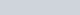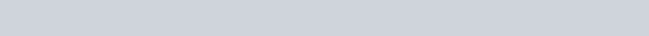Neutralization by Acid Base Titration Lab Report

Name __________________________________

Date ____________________________ Section_________________________________

# INTRODUCTION

A neutralization reaction is a double-displacement reaction in which an acid and base are the reactants and liquid water and an ionic salt are the products:

Acid(aq) + Base(aq) Æ Ionic Salt(aq) + H2O(l)

Recall that an acid is a compound that will release H+ ions into solution, and a base is a compound that will release OH ions into solution. Neutralization reactions can occur with a monoprotic acid (meaning one H+ ion) such as HCl, or with a diprotic acid (meaning two H+ ions) such as H2CO3, or a polyprotic acid (meaning more than two H+ ions) such as H3PO4. The following examples demonstrate an example of each of these reactions:

HCl(aq) + KOH(aq) Æ KCl(aq) + H2O(l)

H2CO3(aq) + 2 NaOH(aq) Æ Na2CO3(aq) + 2 H2O(l)

2 H3PO4(aq) + 3 Ba(OH)2(aq) Æ Ba3(PO4)2(s) + 6 H2O(l)

Often, the concentration of an acid or a base in solution is measured by titration. A titration is a commonly used technique in which a solution with a known concentration is slowly added to a solution of unknown concentration. In the acid-base titration being performed today, an H2SO4 sample of unknown concentration will be titrated with a NaOH solution of known concentration. Using the molarity of NaOH and amount of base added, along with the volume of the H2SO4 solution, the concentration of H2SO4 solution can be determined.

Example Calculation – In an acid-base titration, 50.00 mL of 0.5000 M sodium hydroxide is needed to neutralize 32.26 mL of sulfuric acid. What is the molarity of the aqueous sulfuric acid solution?

Write the neutralization reaction: H2SO4(aq) + 2 NaOH(aq) Æ Na2SO4(aq) + 2 H2O(l)

Recall that molarity (M), is expressed as:

𝑚𝑚𝑚𝑚𝑚𝑚𝑚𝑚𝑚𝑚 𝑚𝑚𝑜𝑜 𝑚𝑚𝑚𝑚𝑚𝑚𝑠𝑠𝑚𝑚𝑚𝑚 𝑚𝑚𝑚𝑚𝑚𝑚𝑚𝑚𝑚𝑚

𝑚𝑚𝑚𝑚𝑚𝑚𝑚𝑚𝑚𝑚𝑚𝑚𝑚𝑚𝑚𝑚 = 𝑚𝑚𝑚𝑚 𝑀𝑀 =

𝑚𝑚𝑚𝑚𝑚𝑚𝑚𝑚𝑚𝑚𝑚𝑚 𝑚𝑚𝑜𝑜 𝑚𝑚𝑚𝑚𝑚𝑚𝑠𝑠𝑚𝑚𝑚𝑚𝑚𝑚𝑠𝑠 𝑉𝑉

To determine the molarity of the sulfuric acid solution, first find the moles of sodium hydroxide added to the solution:

0.5000 𝑚𝑚𝑚𝑚𝑚𝑚

𝑀𝑀𝑉𝑉 = 𝑚𝑚𝑚𝑚𝑚𝑚𝑚𝑚𝑚𝑚 = ∗ 0.05000 𝐿𝐿 = 0.02500 𝑚𝑚𝑚𝑚𝑚𝑚𝑚𝑚𝑚𝑚 𝑁𝑁𝑚𝑚𝑁𝑁𝑁𝑁

𝐿𝐿

13 –

We will then use the stoichiometry of the reaction to calculate the moles of H2SO4 that reacted with the NaOH.

1. 𝑚𝑚𝑚𝑚𝑚𝑚 𝑁𝑁2𝑆𝑆𝑁𝑁4

𝑚𝑚𝑚𝑚𝑚𝑚𝑚𝑚𝑚𝑚 𝑁𝑁2𝑆𝑆𝑁𝑁4 = 0.02500 𝑚𝑚𝑚𝑚𝑚𝑚𝑚𝑚𝑚𝑚 𝑁𝑁𝑚𝑚𝑁𝑁𝑁𝑁 ∗ = 0.01250 𝑚𝑚𝑚𝑚𝑚𝑚 𝑁𝑁2𝑆𝑆𝑁𝑁4

1. 𝑚𝑚𝑚𝑚𝑚𝑚𝑚𝑚𝑚𝑚 𝑁𝑁𝑚𝑚𝑁𝑁𝑁𝑁

We can then calculate the molarity of the sulfuric acid by dividing the moles of H2SO4 by the original volume in liters:

0.0125 𝑚𝑚𝑚𝑚𝑚𝑚 𝑁𝑁2𝑆𝑆𝑁𝑁4

0.03226𝐿𝐿 = 𝟎𝟎. 𝟑𝟑𝟑𝟑𝟑𝟑 𝑴𝑴 𝑯𝑯𝟐𝟐𝑺𝑺𝑶𝑶𝟒𝟒

We can also put all of these steps together for one calculation:

0.. 𝟑𝟑𝟑𝟑𝟑𝟑 𝑴𝑴 𝑯𝑯𝟐𝟐𝑺𝑺𝑶𝑶𝟒𝟒

In today’s experiment you will prepare a standard sodium hydroxide solution. You are going to standardize your NaOH solution by titrating a known mass of potassium hydrogen phthalate (KHP). NaOH and KHP react in a 1:1 ratio. Note that KHP is just an abbreviation and its actual chemical formula is C8H5KO4. You will then slowly and carefully add the standardized NaOH solution to a sample of H2SO4 solution using a burette, a device used to precisely deliver a specific volume of solution. The NaOH will be added until all of the H2SO4 has been consumed. This point is called the equivalence point of the titration, the point where the moles of acid are equal to the moles of base. As both NaOH and H2SO4 are clear and colorless, an indicator must be used to indicate the equivalence point of the titration. An indicator is a chemical that will produce an observable change when a chemical reaction occurs. The indicator used in this experiment is phenolphthalein, a pH sensitive dye that is colorless in acidic solutions and dark pink in basic solutions. At the equivalence point of the titration, adding even one additional drop of the NaOH solution will change the color of the solution in the Erlenmeyer flask from colorless to a very pale pink. The color changes in a titration seen while using phenolphthalein as an indicator are shown in Figure 12.1 below. The indicator must be added before the titration begins.Figure 12.1 – Titration Color Changes with Phenolphthalein as an IndicatorUnless otherwise noted, content of Santa Monica College is licensed under CC BY 4.0 PROCEDURE:

Part A – Preparation and Standardization of NaOH(aq) (simulation)

1. Navigate to the following video that describes how to set up a buret.

Watch this video for directions on reading a buret:

This last video shows the general steps in a titration:

1. Now navigate to the Standardization of NaOH with a KHP solution simulation available from ChemCollective at http://chemcollective.org/vlab/101.

1. From the stockroom, obtain:
• 0.1 L of the NaOH solution of unknown concentration
• 25 mL of a 0.500 M KHP solution
• a solution of phenolphthalein
• a 50 mL burette (located under ‘other’ glassware).

The stockroom is located on the left side of the simulation. To choose glassware, solutions, or tools, click on the appropriate tab. To add something to your lab bench, click on the ‘+’ icon for the item. To rename or remove something from the bench, right click on the item.

1. Fill the burette with the NaOH solution by dragging the Erlenmeyer flask containing NaOH on top of the burette. A dialog box will pop up. Choose precise mode. You will pour 52.00 mL into the box and clicking ‘pour’. This will enough volume to give a starting volume of 0.00 mL. Record the initial volume in the burette in Data Table 1.

1. Add 1.00 mL of phenolphthalein indicator to the flask containing 0.500 M KHP.

1. Drag the burette on top of, and literally into, the flask that contains 0.500 M KHP to start the titration. This is tricky. A dialogue box pop-up will indicate if you were successful. In the dialog box, select ‘realistic’ and hold down the left mouse button to open the burette and titrate the KHP solution.

1. As you add the NaOH the color of the receiving solution (called the analyte) will eventually turn pink. To check your progress, slow the NaOH addition and visually inspect the color of the receiving solution. On the first trial, it’s very likely that you will overshoot the endpoint because you had no initial estimate of the volume of titrant needed. Rather than record this 1st volume, use it as a rough estimate of the endpoint volume.

1. Discard the flask containing the titrated KHP by right clicking on it and selecting ‘Remove’. From the stockroom, obtain another flask containing 25 mL of 0.500 M KHP. Add 1 mL of phenolphthalein to the flask.

1. Titrate the new analyte with the NaOH solution from the burette. This time slow the titration as you approach the endpoint volume from Step 7. Record the endpoint volume for the titration when the analyte solution turns pale pink.

1. Repeat steps 8-9 to obtain two more endpoint volumes.

1. Discard all solutions from the lab bench and complete the Data Analysis section for Part 1.

1. Calculate the molarity of the NaOH solution for each trial and then record the average molarity of NaOH in Data Table 1.

Data Table 1. Part A – Preparation and Standardization of NaOH(aq)

 Trial 1 Trial 2 Trial 3 Volume of 0.500 M KHP used (mL) 25.00 mL 25.00 mL 25.00 mL Initial Burette Reading (mL) Final Burette Reading (mL) Volume of NaOH used (Vf – Vi) (mL)

Part B – Concentration of H2SO4(aq) (‘dry’ lab data)

1. After standardizing the sodium hydroxide solution in Part A, you used it to titrate three H2SO4 (aq) samples. Each sample was prepared by using a 10.00 mL volumetric pipette to transfer exactly 10.00 mL of H2SO4 (aq) to each of the volumetric flasks and 20 mL of distilled water was added to the Erlenmeyer flask with 3-4 drops of phenolphthalein indicator.

1. Use the titration results in the Data Table 2 (below) to calculate the molarity of H2SO4 for each of the three titrations and report the average molarity of H2SO4 in Data Table 2. Part B.

Data Table 2. Part B – Concentration of H2SO4 (aq)

 Trial 1 Trial 2 Trial 3 Volume of H2SO4 used (mL) 10.00 mL 10.00 mL 10.00 mL Initial Burette Reading (mL) 0.00 mL 7.80 mL 15.95 mL Final Burette Reading (mL) 7.80 mL 15.95 mL 24.00 mL Volume of NaOH used (Vf – Vi) (mL)

# DATA ANALYSIS

1. Determine the molarity of NaOH for each of the three trials. Remember that NaOH and KHP (C8H5KO4) react in a 1:1 ratio. Show all your work for full credit.

1. What is the average molarity of NaOH from the three trials?

1. Write a complete and balanced chemical equation for the reaction between NaOH and H2SO4.

1. Determine the molarity of the H2SO4 for each of the three trials using the average molarity of NaOH that you calculated in #2. Show all your work for full credit.

1. What is the average molarity of H2SO4 from the three trials?

1. Obtain the molarity of H2SO4 from your instructor. Find the percent error of your titrations.

1. Name two sources of error in this experiment. Explain how each of these errors could have affected the molarity of H2SO4.

# POST-LABORATORY QUESTIONS

1. Write the balanced chemical equation for the neutralization of hydrobromic acid with a solution of calcium hydroxide.

1. A sample of solid sodium hydroxide, weighing 13.20 grams is dissolved in deionized water to make a solution. What volume in mL of 0.235 M H2SO4 will neutralize this solution? Write the balanced equation for the chemical reaction first.

1. What volume in milliliters of 0.250 M HNO2 is required to neutralize 36.0 milliliters of a 0.150 M NaOH solution

1. How many grams of calcium hydroxide, Ca(OH)2 are required to neutralize 43.26 milliliters of 0.550 M H2SO4 solution?

# PRE-LABORATORY ASSIGNMENT

1. In the experiment for today, which is solution is added to the burette, and which solution is added to the Erlenmeyer flask in Part A? What about in Part B? Label the solutions in the figure below for Part B.1. Is the concentration of the base known or unknown in Part A? Is the concentration of the acid known or unknown in Part A?

1. Is the concentration of the base known or unknown in Part B? Is the concentration of the acid known or unknown in Part B?

1. What is the equivalence point? How will you know when you have reached it in today’s experiment?

1.What indicator are we using in today’s experiment? At what point in the experiment do you add the indicator? How much indicator is needed?

1. If you start with a sample of solid potassium KOH, weighing 12.5 grams, what volume in mL of a

0.500 M HCl solution will neutralize this solution? Write the balanced neutralization reaction first.